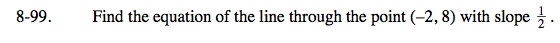### Home > CAAC > Chapter 8 > Lesson 8.3.1 > Problem8-99

8-99.

Find the equation of the line through the point (−2, 8) with slope. Homework Help ✎The equation of a line is y = mx + b.

m = growth (slope)
b = starting point (y-intercept)

Substitute the points from the problem into the equation. Solve for b.